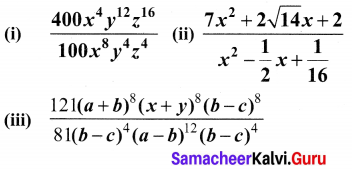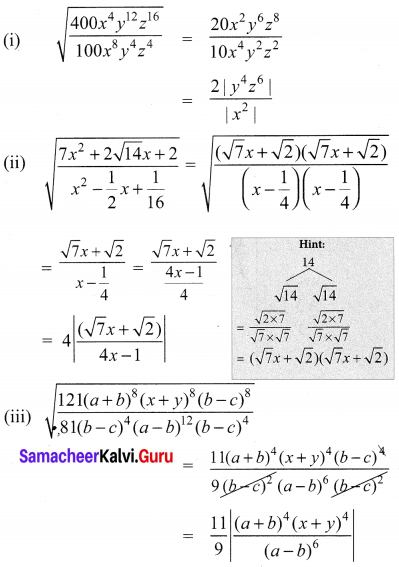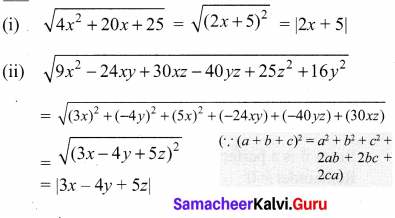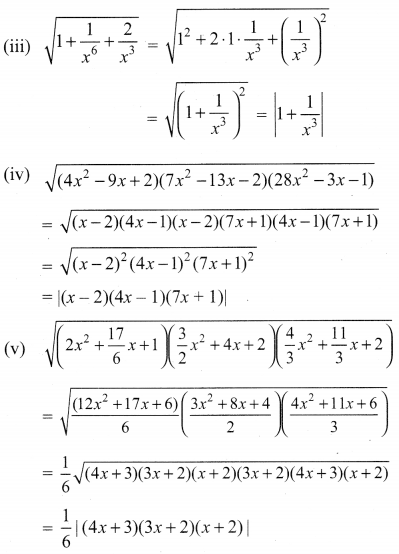## Tamilnadu Samacheer Kalvi 10th Maths Solutions Chapter 3 Algebra Ex 3.7

10th Maths Exercise 3.7 Samacheer Kalvi Question 1.
Find the square root of the following rational expressions.Solution:Ex 3.7 Class 10 Samacheer Question 2.
Find the square root of the following
(i) 4x2 + 20x + 25
(ii) 9x2 – 24xy + 30xz – 40yz + 25z2 + 16y2
(iii) 1 + $$\frac{1}{x^{6}}+\frac{2}{x^{3}}$$
(iv) (4x2 – 9x + 2) (7x2 – 13x – 2) (28x2 – 3x – 1)
(v) ( 2x2 + $$\frac{17}{6} x$$ + 1) ($$\frac{3}{2} x^{2}$$ + 4x + 2)($$\frac{4}{3} x^{2}+\frac{11}{3} x$$ + 2)
Solution: